•••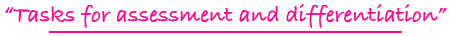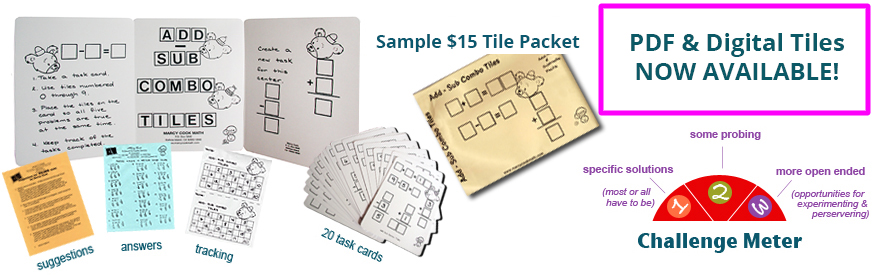Tiling Task Card Packets include 20 unique task cards on card stock, 3 backdrop cards, tracking sheets, suggestions and answer sheets.  These are contained in a printed manila envelope for convenient storing.  A set of number tiles 0 - 9 is  needed for each task card.

4 - 7

### MULTIPLICATION/DIVISION TILES

completing multiplication and division equations and dealing with factors of numbers and multiples of numbers

Challenge Level: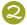\$15.00

NEW
4 - 6

### MULTIPLY TO A PRODUCT TILES

forming basic multiplication facts with 2 or 3 factors to a given product

Challenge Level:AVAILABLE IN PDF FORMAT ONLY
(You will receive both color and black and white files for printing.)\$10.00

5 - 8

### MULTIPLY: THINK & TILE

forming a multiplication problem with a 4-digit number as a factor and a single-digit number as a factor by utilizing hints dealing with math concepts and vocabulary

Challenge Level: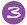\$15.00

5 - 8

### MULTIPLY: TRIAL & TILE

forming a multiplication problem where a 3-digit number is multiplied by a 2-digit number

Challenge Level:\$15.00

3 - 6

### PROBLEM PRODUCT TILES

experimenting with digits to form multiplication fact problems and products

Challenge Level:\$15.00

4 - 8

### TARGET NUMBER MULTIPLY TILES

discovering different ways to form a specified number utilizing the operation of multiplication

Challenge Level:\$15.00

3 - 6

### TIMES TABLE TILES

open experimenting with digits 0 through 9 to form 3 different multiplication problems/products

Challenge Level:\$15.00

4 - 8

### 2 X 1 MULTIPLICATION TILES

multiplying 2-digit numbers by a single-digit number to yield a 2-digit or 3-digit product

Challenge Level:\$15.00

1 - 3

### X PICTURE X TILES

counting to show multiplication facts in pictorial arrays; products to 21

Challenge Level: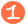\$15.00